# Learn you Galois Fields for Great Good (01)

Navigation | first | previous | next

## Group Theory

This is part 1 of a series on Abstract Algebra. In this part, we'll give a gentle introduction to Group Theory.

### Clock Arithmetic

A Group is one of the basic algebra structures studied in Abstract Algebra. It's simply a set of "numbers" and an "operation" on them. The operation is normally referred to as "addition" or "multiplication" but the operation may behave somewhat differently than what you're used to.

In fact, you are already very familiar with a Group: A Clock.

Of course, as computer scientists, we must start our numbers at 0 because we're all neurodivergent and we take great pleasure in annoying mathematicians.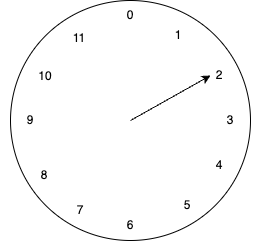Our clock uses the numbers `{0, 1, 2, 3, 4, 5, 6, 7, 8, 9, 10, 11}` and has the operation of `"an hour passes"`. If the hour-hand points to 2 and the "operation" occurs, it now points at 3.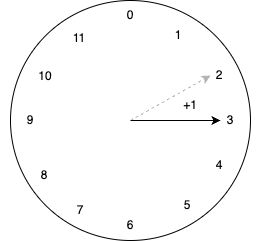Similairly, if it points at 11, then after the "operation" it will point to 0 (rolls over to the beginning).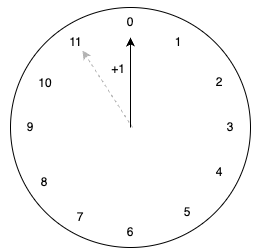Or we can "add" 5 hours to 8 and we will get 1.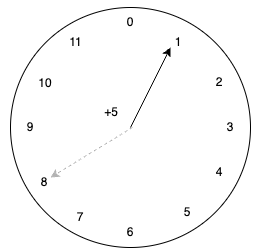Or we can "add" 0 hours and the hour will stay the same!

We can even "subtract", which is going backwards around the clock. Subtracting 5 hours from 8 gives 3. Just like arithmetic over integers, subtraction is just addition in disguise. We just add the "negative". If we want to subtract 5, we could instead add 7:

``````8 - 5 = 8 + (-5) = 8 + 7 = 3
``````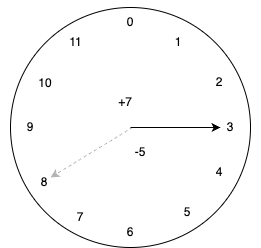In short, we can do all of the following on this clock:

• We can `add n hours` to any position, yielding a new position on the clock
• We can find an `additive-inverse` of any position that can convert any subtraction into an addition
• We can add `zero` and the position doesn't change
• We can do `associative` addition, i.e. (1 + 2) + 3 = 1 + (2 + 3)

Exercise: Work through some examples and convince yourself of these facts. Internalizing these ideas is critical to keeping your sanity as things get more abstract.

Here are some examples of operations on our clock. Work through each to make sure you understand completely. Try your own examples. I'm serious. We are going to generalize / abstract this significantly and it helps to have a very solid understanding of a concrete example.

a b -b a + b a - b a + -b
5 0 0 5 5 5
1 1 11 2 0 0
4 3 9 7 1 1
11 2 10 1 9 9
6 6 6 0 0 0

### Group definition

The clock example we gave above is an example of a Group. It's helpful to keep the example in mind as we start introducing abstract definitions.

A Group is defined as some operation (`+`) defined over a set of `"numbers"` which follow these rules:

1. Associativity: `(a + b) + c = a + (b + c)`
2. Identity: one of the numbers `e` acts like a `"zero"`: `a + e = a, e + a = a`
3. Inverse: each element `a` has some unique element `b` where `a + b = e`

And that's it.

The name of the operation isn't important. Sometimes we use the multiplication (`*`) operator instead. The notation doesn't matter.

Exercise: Convince yourself that our clock is a group according to these definitions.

### Modular Arithmetic

Our clock arithmetic group is an example of a Modular Arithmetic. In particular, it's the special case where `modulus n = 12`. There's no special reason we need to use 12. We can use any `modulus n` we like. Over the operation of addition, this always forms a group!

In modular arithmetic, the following notation is commonly used:

``````a == b (mod n)
``````

This says that `a` and `b` are logically the same value when using `modulus n`. Consider our clock again, if the hour hand points at `5` and we add `12 hours`, we'd be at `17` right? But we don't have a 17 hour hand. Instead we roll over to 5 again.

In modular arithmetic notation, we'd express this as:

``````17 == 5 (mod 12)
``````

Similairly there are an infinite number of integers that are "equivalent" to 5 when `modulus n=12`:

``````5 == 5 (mod 12)
17 == 5 (mod 12)
29 == 5 (mod 12)
41 == 5 (mod 12)
53 == 5 (mod 12)
(... etc ...)
``````

In general:

``````5 + k*12 == 5 (mod 12)   [for any integer k]
``````

In this way, we can think of modular arithmetic as doing normal arithmetic and then only keeping the remainder after applying the modulus.

Exercise: Play around with the modular arithmetic where `n=5` (a) what are the numbers? (b) which number acts as an identity? (c) what is the "additive inverse" of 3? (d) which integers are "equivalent" to 1?

Do the exercise. Each section gets harder. You need to fully understand each or you'll get lost later.

### Modular Arithmetic: Multiplication

We want to do:

``````a * b = c (mod n)
``````

Examples with our n=12 clock:

``````5 * 4 = 20 == 8 (mod 12)
3 * 2 = 6 == 6 (mod 12)
``````

Exercise: Does this form a Group? Justify each required property or give a counter-example.

Don't read on before completing the exercise.

...

...

...

First, it's clear that 1 must be the identity element because:

``````a * 1 = 1 * a = a (mod 12)
``````

We need a multiplicative inverse for each element, including 0:

``````0 * a = 1
``````

But, there's no solution! So the set `{0, 1, 2, 3, 4, 5, 6, 7, 8, 9, 10, 11}` is not a group using multiplication.

What if we just get rid of 0 then and use the subset `{1, 2, 3, 4, 5, 6, 7, 8, 9, 10, 11}` ?

Is this a group now? Unfortunately no. Not every element has an "inverse".

Consider the element `2`. We need to find some `x` such that: `2 * x = 1`.

Exercise: Try each of the numbers `{1, 2, 3, 4, 5, 6, 7, 8, 9, 10, 11}` for `x` to try solving `2 * x = 1`. Is there an inverse for `2`? Can you think of simple reason why not?

So maybe modular arithmetic doesn't work? Give Up? Actually no.

Modular arithmetic over multiplication works if the modulus n is prime. This is a direct result of important facts in number theory involving the Greatest Common Denominator and Bezout's Identity.

Proof (you can skip this):

Let `a` be some number from the set of numbers in our Group. And suppose we wish to find an inverse `b` such that:

``````b * a == 1 (mod n)
``````

We'll rearrange this expression:

``````b * a = 1 + k*n        [for some constant k: 1 + k*n == 1 (mod n) for all k]
b * a + (-k) * n = 1   [move n to left-side]
``````

We've achieved a form where we can use an important identity.

Bezout's Identity states that given `x` and `y`, we can always solve for `a` and `b` in the equation:

``````  a*x + b*y = gcd(x, y)
``````

So we can solve:

``````b * a + (-k) * n = gcd(a, n)
``````

And `b` will be our desired inverse when:

``````gcd(a, n) = 1
``````

When `n` is prime, we have `gcd(a, n) = 1` always. So we must always have a solution.

The proof is complete.

With this result we can satisfy our 3 group properties and conclude that modular-arithmetic over the sets `{1, 2, ..., p-1}` is always a group when `p` is prime.

Exercise: Explore n=5 modular-arithmetic over multiplication using the set {1, 2, 3, 4}: (a) draw the multiplication table (it should have 16 squares), (b) draw the inverse table (it should have 4 squares)

Exercise: Explore n=4 modular-arithmetic over multiplication using the set {1, 2, 3}: (a) give a counter-example for why this is not a Group

### Next Steps

You should be very comfortable with this material before moving on. If you aren't, go back and do more examples. Perhaps draw some clocks with different number of hours and see what happens? This is a very cumulative subject and in each section, I will assume you've mastered the previous section. If you don't master it, you will eventually get very lost.

Next up, we will extend Group Theory into Field Theory.

Let's do it